# Logical Reasoning and Analytical Ability (Test 2)

## Upsc Civil Services Entrance Exams : General Studies Test

| Home | | Upsc Civil Services Entrance Exams | | General Studies Test | | Logical Reasoning and Analytical Ability |Logical Reasoning and Analytical Ability
| Logical Reasoning | | Analytical Reasoning |
Q.1
Find the minimum number of straight lines required to make the given figure.A. 16
B. 17
C. 18
D. 19
Explaination / Solution:

The figure may be labelled as shown.The horizontal lines are IK, AB, HG and DC i.e. 4 in number.

The vertical lines are AD, EH, JM, FG and BC i.e. 5 in number.

The slanting lines are IE, JE, JF, KF, DE, DH, FC and GC i.e. 8 is number.

Thus, there are 4 + 5 + 8 = 17 straight lines in the figure.

Workspace
Report
Q.2
Find the number of triangles in the given figure.A. 16
B. 22
C. 28
D. 32
Explaination / Solution:

The figure may be labelled as shown.The simplest triangles are AFJ, FJK, FKB, BKG, JKG, JGC, HJC, HIJ, DIH, DEI, EIJ and AEJ i.e. 12 in number.

The triangles composed of two components each are JFB, FBG, BJG, JFG, DEJ, EJH, DJH and DEH i.e. 8 in number.

The triangles composed of three components each are AJB, JBC, DJC and ADJ i.e. 4 in number.

The triangles composed of six components each are DAB, ABC, BCD and ADC i.e. 4 in number.

Thus, there are 12 + 8 + 4 + 4 = 28 triangles in the figure.

Workspace
Report
Q.3
Find the number of triangles in the given figure.A. 10
B. 19
C. 21
D. 23
Explaination / Solution:

The figure may be labelled as shown.The simplest triangles are ABI, BIC, AIJ, CIJ, AHJ, CDJ, JHG, JDE, GJF and EJF i.e. 10 in number.

The triangles composed of two components each are ABC, BCJ, ACJ, BAJ, AJG, CJE and GJE i.e. 7 in number.

The triangles composed of four components each are ACG, ACE, CGE and AGE i.e. 4 in number.

Total number of triangles in the figure =10+ 7 + 4 = 21.

Workspace
Report
Q.4

Find the number of triangles in the given figure.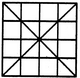A. 36
B. 40
C. 44
D. 48
Explaination / Solution:

The figure may be labelled as shown.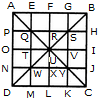The simplest triangles are APQ, AEQ, QTU, QRU, BGS, BHS, RSU, SUV, TUW, UWX, NWD, WDM, UVY, UXY, JCY and YKC i.e. 16 in number.

The triangles composed of two components each are QUW, QSU, SYU and UWY i.e. 4 in number.

The triangles composed of three components each are AOU, AFU, FBU, BIU, UIC, ULC, ULD and OUD i.e. 8 in number.

The triangles composed of four components each are QYW, QSW, QSY and SYW i.e. 4 in number.

The triangles composed of six components each are AUD, ABU, BUC and DUC i.e. 4 in number.

The triangles composed of seven components each are QMC, ANY, EBW, PSD, CQH, AGY, DSK and BJW i.e. 8 in number.

The triangles composed of twelve components each are ABD, ABC, BCD and ACD i.e. 4 in number.

Thus, there are 16 + 4 + 8 + 4 + 4 + 8 + 4 = 48 triangles in the figure.

Workspace
Report
Q.5

Find the number of triangles in the given figure.A. 18
B. 20
C. 28
D. 34
Explaination / Solution:

The figure may be labelled as shown.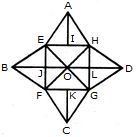The simplest triangles are AEI, AIH, BEJ, BJF, CFK, CKG, DGL, DLH, EOJ, FOJ, FOG, LOG, HOL and HOE i.e. 14 in number.

The triangles composed of two components each are EAH, FBE, BEO, EOF, BFO, FCG, GDH, HOD, HOG and GOD i.e. 10 in number.

The triangles composed of three components each are EFH, EHG, FGH and EFG i.e. 4 in number.

Thus, there are 14 + 10 + 4 = 28 triangles in the given figure.

Workspace
Report
Q.6
What is the number of triangles that can be formed whose vertices are the vertices of an octagon but have only one side common with that of octagon?
A. 64
B. 32
C. 24
D. 16
Explaination / Solution: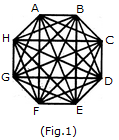When the triangles are drawn in an octagon with vertices same as those of the octagon and having one side common to that of the octagon, the figure will appear as shown in (Fig. 1).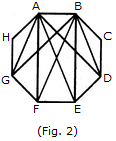Now, we shall first consider the triangles having only one side AB common with octagon ABCDEFGH and having vertices common with the octagon (See Fig. 2).Such triangles are ABD, ABE, ABF and ABG i.e. 4 in number.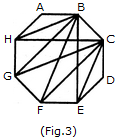Similarly, the triangles having only one side BC common with the octagon and also having vertices common with the octagon are BCE, BCF, BCG and BCH (as shown in Fig. 3). i.e. There are 4 such triangles.

This way, we have 4 triangles for each side of the octagon. Thus, there are 8 x 4 = 32 such triangles.

Workspace
Report
Q.7

Count the number of squares in the given figure.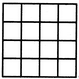A. 32
B. 30
C. 29
D. 28
Explaination / Solution:

The figure may be labelled as shown.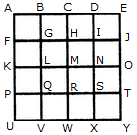The simplest squares are ABGF, BCHG, CDIH, DEJI, FGLK, GHML, HINM, IJON, KLQP, LMRQ, MNSR, NOTS, PQVU, QRWV, RSXW and STYX i.e. 16 in number.

The squares composed of four components each are ACMK, BDNL, CEOM, FHRP, GISQ, HJTR, KMWU, LNXV and MOYW i.e. 9 in number.

The squares composed of nine components each are ADSP, BETQ, FIXU and GJYV i.e. 4 in number.

There is one square AEYU composed of sixteen components.

There are 16 + 9 + 4 + 1 = 30 squares in the given figure.

Workspace
Report
Q.8

Count the number of triangles and squares in the given figure.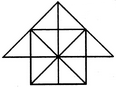A. 26 triangles, 5 squares
B. 28 triangles, 5 squares
C. 26 triangles, 6 squares
D. 28 triangles, 6 squares
Explaination / Solution:

The figure may be labelled as shown.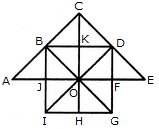Triangles:

The simplest triangles are JBO, BKO, KDO, DFO, FGO, GHO, HIO, IJO, ABJ, BCK, CKD and DEF i.e.12 in number.

The triangles composed of two components each are IBO, BDO, DGO, GIO, ABO, CDO, CBO, CBD and DEO i.e. 9 in number.

The triangles composed of four components each are IBD, BDG, DGI, GIB, ACO and COE i.e. 6 in number.

There is only one. triangle i.e. ACE composed of eight components.

Thus, there are 12 + 9 + 6 + 1 = 28 triangles in the given figure.

Squares:

The squares composed of two components each are BKOJ, KDFO, OFGH and JOHI i.e. 4 in number.

There is only one square i.e. CDOB composed of four components.

There is only one square i.e. BDGI composed of eight components.

Thus, there are 4 + 1 + 1 = 6 squares in the given figure.

Workspace
Report
Q.9

Count the number of triangles and squares in the given figure.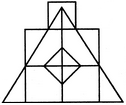A. 21 triangles, 7 squares
B. 18 triangles, 8 squares
C. 22 triangles, 7 squares
D. 20 triangles, 8 squares
Explaination / Solution:

The figure may be labelled as shown.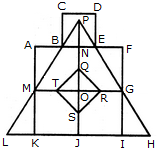Triangles :

The simplest triangles are BPN, PNE, ABM, EFG, MLK, GHI, QRO, RSO, STO and QTO i.e. 10 in number.

The triangles composed of two components each are BPE, TQR, QRS, RST and STQ i.e. 5 in number.

The triangles composed of three components each are MPO and GPO i.e. 2 in number.

The triangles composed of six components each are LPJ, HPJ and MPG i.e. 3 in number.

There is only one triangle LPH composed of twelve components.

Total number of triangles in the figure = 10 + 5 - 2 + 3 + 1 = 21.

Squares :

The squares composed of two components each are KJOM and JIGQ i.e. 2 in number.

The squares composed of three components each are ANOM, NFGO and CDEB i.e.3 in number.

There is only one square i.e. QRST composed of four components.

There is only one square i.e. AFIK composed of ten components.

Total number of squares in the figure = 2 + 3 + 1 + 1 = 7.

Workspace
Report
Q.10

Count the number of convex pentagons in the adjoining figure.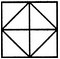A. 16
B. 12
C. 8
D. 4
Explaination / Solution:

A convex pentagon has no angles pointing inwards. More precisely, no internal angles can be more than 180°.

The figure may be labelled as shown.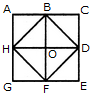The pentagons in the figure, are ABDFH, CDFHB, EFHBD, GHBDF, ACDFG, CEFHA, EGHBC, GABDE, BDEGH, DFGAB, FHACD and HBCEF. Clearly, these are 12 in number.

Workspace
Report